Courses

# Ex 8.1 NCERT Solutions- Introduction to Trigonometry Class 10 Notes | EduRev

## Class 10 : Ex 8.1 NCERT Solutions- Introduction to Trigonometry Class 10 Notes | EduRev

The document Ex 8.1 NCERT Solutions- Introduction to Trigonometry Class 10 Notes | EduRev is a part of the Class 10 Course Mathematics (Maths) Class 10.
All you need of Class 10 at this link: Class 10

Q.1. In Δ ABC, right-angled at B, AB = 24 cm, BC = 7 cm. Determine:
(i) sin A, cos A
(ii) sin C, cos C
Sol. In right angled Δ ABC,
AC2 = AB2 + BC2
[Pythagoras Theorem]
AC2 = (24)2 + (7)2
= 576 + 49 = 625
⇒ AC = 25 cm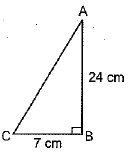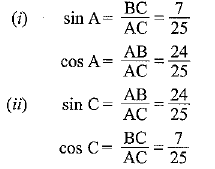Q.2. In the figure, find tan P − cot R.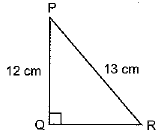Sol. In right angled ΔPQR,
PR2 = PQ2 + QR2
[Pythagoras Theorem]
⇒ (13)2 = (12)2 + QR2
⇒ 169 - 144 = OR2
⇒ 25 = OR2 ⇒ OR = 5 cm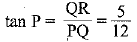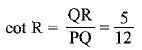So,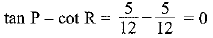Q.3. If sin A = 3/4, calculate cos A and tan A.
Sol.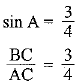Let BC = 3k
and AC = 4k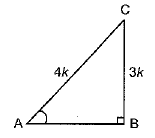By Pythagoras theorem,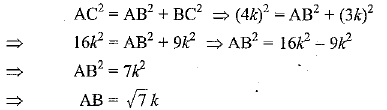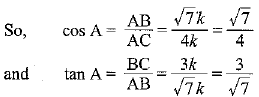Q.4. Given 15 cot A = 8, find sin A and sec A.
Sol. 15 cot A = 8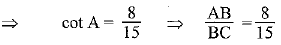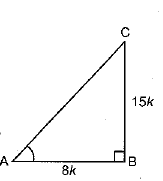Let AB = Sk and BC = 15 k
In right angled ΔABC,
AC2 = AB2 + BC2
[Pythagoras theorem]
= (8k)2 + (15k)2
= 64k2 + 225k2 = 289k2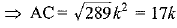So,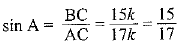and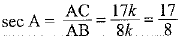Q.5. Given sec θ = 13/12, calculate all other trigonometric ratios.
Sol.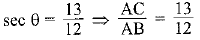Let AC = 13k and AB = 12k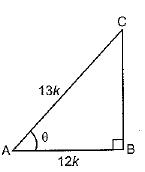In right angled Δ ABC,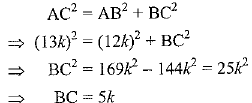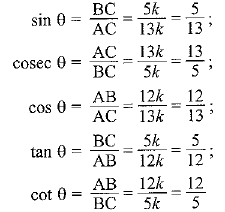Q.6. If ∠A and ∠B are acute angles such that cos A = cos B, then show that ∠A = ∠B.
Sol. Since A and B are acute angles.
Then, C = 90°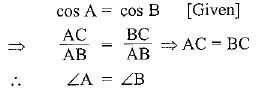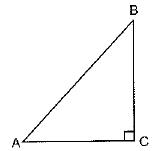[Angles opposite to equal sides are equal]

Q.7. If cot θ = 7/8, evaluate: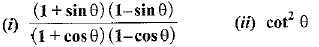Sol. cot θ = 7/8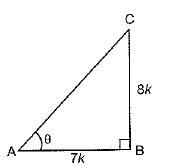In right angled ΔABC, AB/BC = 7/8
Let, AB = 7k and BC = 8k
In right angled ΔABC, AC2 = AB2 + BC2
[Pythagoras theorem]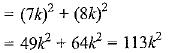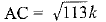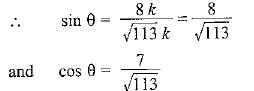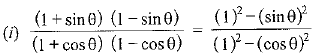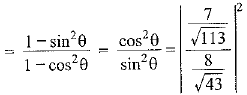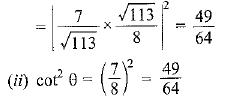Q.8. If 3 cot A = 4, check whether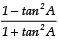= cos2 A − sin2 A or not.
Sol. 3 cot A = 4 [Given]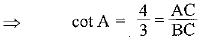Let AC = 4k and BC = 3k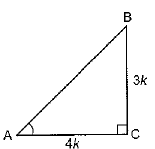In right angled ∆ACB,
AB2 = AC2 + BC2
= (4k)2 + (3k)2 = 16k2 + 9k2 = 25k2
⇒ AB = 5k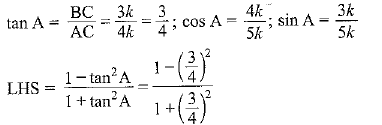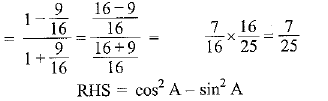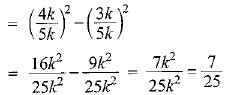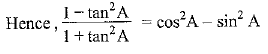Q.9. In triangle ABC, right-angled at B, if tan A =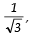find the value of:
(i) sin A cos C + cos A sin C
(ii) cos A cos C − sin A sin C
Sol.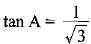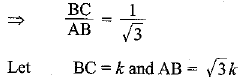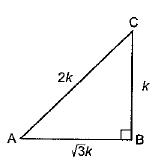In right angled ΔABC,
AC2 = AB2 + BC2    [Pythogoras theorem]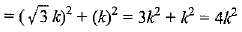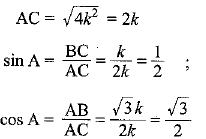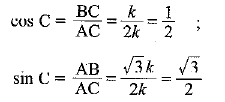(i) sin A cos C + cos A sin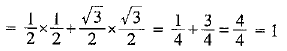(ii) cos A cos C - sin A sin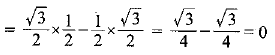Q.10. In ΔPQR, right-angled at Q, PR + QR = 25 cm and PQ = 5 cm. Determine the values of sin P, cos P and tan P.
Sol.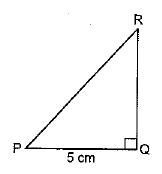In right angled ΔPQR
PR2 = PQ2 + QR2
⇒ PQ2 = PR2 = QR2
⇒ (5)2 = (PR + QR) (PR - QR)
⇒ 25 = 25 (PR - QR)
⇒ 25/25 = PR - OR
⇒ PR - QR = 1  .... (i)
and  PR + QR = 25    ... (ii)
On adding equation (i) and (ii), we get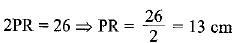From equation (i),
PR - QR = 1 ⇒ QR = 13 - 1 = 12 cm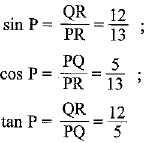Q.11. State whether the following are true or false. Justify your answer.
(i) The value of tan A is always less than 1.
(ii) sec A = 12/5 for some value of angle A.
(iii) cos A is the abbreviation used for the cosecant of angle A.
(iv) cot A is the product of cot and A.
(v) sin θ = 4/3 for some angle θ.
Sol. (i) ∵ tan 60° = √3 , Since √3 > 1. (False)
(ii) sec A is always > 1. (True)
(iii) cos A is the abbreviation for cosine A. (False)
(iv) cot without ∠A is meaningless. (False)
(v) sin θ can never be greater than 1.
∵ sin θ = P/H, hypotenuse is always greater than other two sides.
Hence, the given statement is false.

Offer running on EduRev: Apply code STAYHOME200 to get INR 200 off on our premium plan EduRev Infinity!

,

,

,

,

,

,

,

,

,

,

,

,

,

,

,

,

,

,

,

,

,

;